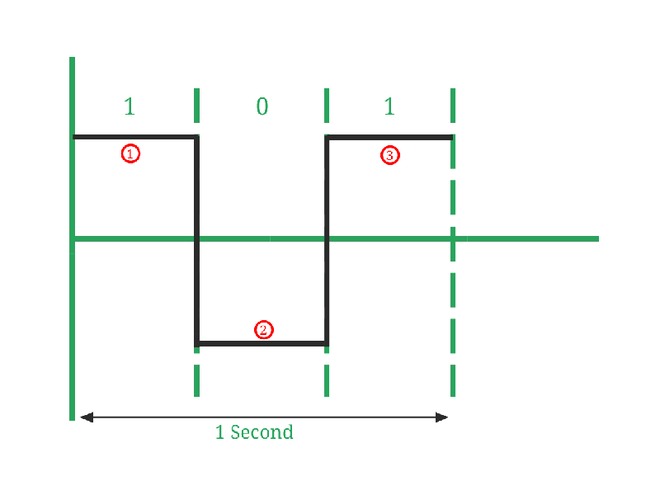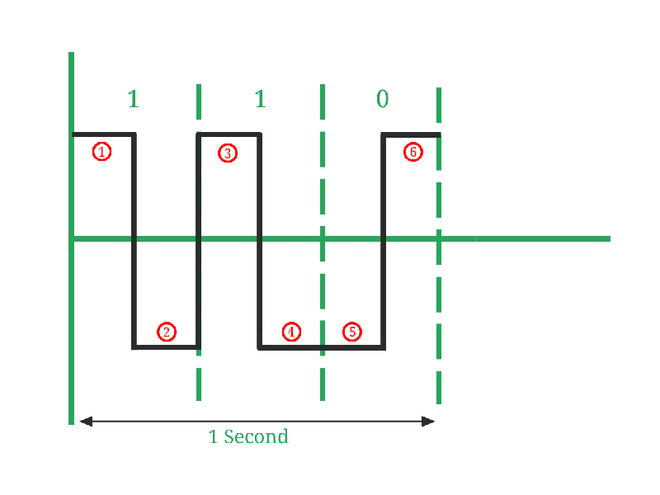Open in App
Not now

# Baud Rate and its importance

• Last Updated : 19 Oct, 2021

Computers communicate by transmitting bits of digital data from one device to another device through transmission media. You can send and receive data without worrying about setting up the details. However, for some devices, we need to supply baud rates and other details.

Old devices use parallel and serial communication ports, though their speeds would be considered slow in today’s time. All the devices that are used today handle all the coordination of communication in the background of the computer.

For example, when you plug a new device in your computer, it prompts a message like “installing device drivers” after they are installed and the device is configured you never have to repeat this process. Though for industrial devices it could be different they might ask you to provide information such as Baud rate, communication ports to route the information, etc.

People often confuse baud rate with bit rate, though they are completely different. The baud rate can be higher or lower than the bit rate depending upon the type of encoding scheme used (Such as NRZ, Manchester, etc.).

1. Bit rate :
Bit rate is the number of binary bits (1s or 0s) transmitted per second.

`Bit rate = number of bits transmitted/ total time (in seconds)`

The bit rate can also be defined in terms of baud rate:

`Bit rate = Baud rate x bits per signal or symbol`

2. Baud rate :
Baud rate is the rate at which the number of signal elements or changes to the signal occurs per second when it passes through a transmission medium. The higher a baud rate is the faster the data is sent/received.

`Baud rate = number of signal elements/total time (in seconds)`

For Example:Image 1

• In Image 1, Number of signal elements (marked in red color) = 3, Number of bits transmitted (1, 0, 1) = 3. So, Here Bit rate = 3/1 = 3 bits per second. And, Baud rate = 3/1 = 3 baud per second.Image 2

• In Image 2, Number of signal elements (marked in red color) = 6, Number of bits transmitted (1, 1, 0) = 3. So, Here Bit rate = 3/1 = 3 bits per second. and, Baud rate = 6/1 = 6 baud per second.

Signal element: A signal element is the smallest unit of a digital signal. A signal is one of several voltages, phase changes, or frequencies. For Digital signals 1 signal element is a signal with constant amplitude. For Analog signals, 1 signal element is a signal with the same amplitude, phase, and frequency.

Why baud rate is important ?
Baud rate is important because:

• Baud rate can determine the bandwidth requirements for transmission of the signal.
• Baud rate is also used for the calculation of the Bit rate of a communication channel.
• It is a tuning parameter (i.e., it adjusts the Network congestion in data networking) for the transmission of a signal.
• It specifies how fast data can be sent over a serial line or serial interface (it’s an interface that sends data as a series of bits over a single wire.).
My Personal Notes arrow_drop_up